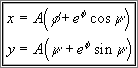### Rogowski profiles

There are applications which require a uniform electrical field between two electrodes such as breakdown voltage testing and large volume laser discharge cavities. The ideal infinite flat plates are somewhat difficult to realize in a lab of reasonable dimensions. Finite sized plates produce a uniform field at the middle of the plate, but the high field at the edges creates a problem.

Rogowski (also variously spelled Rogovski, which is closer to the Russian pronounciation) developed a technique that starts by determining a realizable field, then constructing an electrode shaped so that the surface of the electrode lies on an equipotential surface. He started with an analytical solution of the field due to a finite plane plate parallel to an infinite plane. A more empirical approach was taken by Bruce, described elsewhere.

Taken to an extreme, the field at a large (d>>x) distance from any charge distribution approximates the field from a point charge, i.e. a sphere. The arbitrary charge could be replaced by a sphere of appropriate size and voltage/charge, and the E field would be the same.

For example, consider the field between two finite flat plates. In the center region, the field is quite uniform. However, if one actually constructed such electrodes, you would find that the high field strength at the edges of the plates would almost certainly cause problems from dielectric breakdown. The solution is to construct electrodes that follow an equipotential surface which is some distance from the flat plate electrode. Since it is farther away, the field strength is lower, and breakdown is not as much of an issue.

For an arbitrary shaped electrode, determining the electric field, particularly in the dynamic case, is quite difficult, and would normally need to be done by numerical techniques. However, for some special cases, that happen to be useful, an analytical expression for the field can be developed. Such a case would be the finite flat plates above.If psi <= 0.4pi, the field is greatest in the center region between the plates, and less everywhere else. If you make the electrode follow this contour, the breakdown voltage between the electrodes will be the same as if they were an infinite uniform field.

For the special case of psi = pi/2, the equations above reduce to:Copyright 1998, Jim Lux / rogowski.htm / 24 Jan 1998 / Back to HV Home / Back to home page / Mail to Jim